# Parametric Gears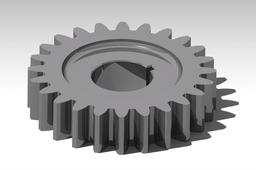In this tutorial I will show you how to make various parametric gears.
You will need basic knowledge of Catia tools.

Property of Feri Čiradžija, mechanical engineer.

1. ### Step 1: Inserting formulas

Catia V5-->Start-->Mechanical design-->Part Design and call it ˝Gear Parametric˝

It is best to press Ctrl+S at the beginning and save your file where you want it.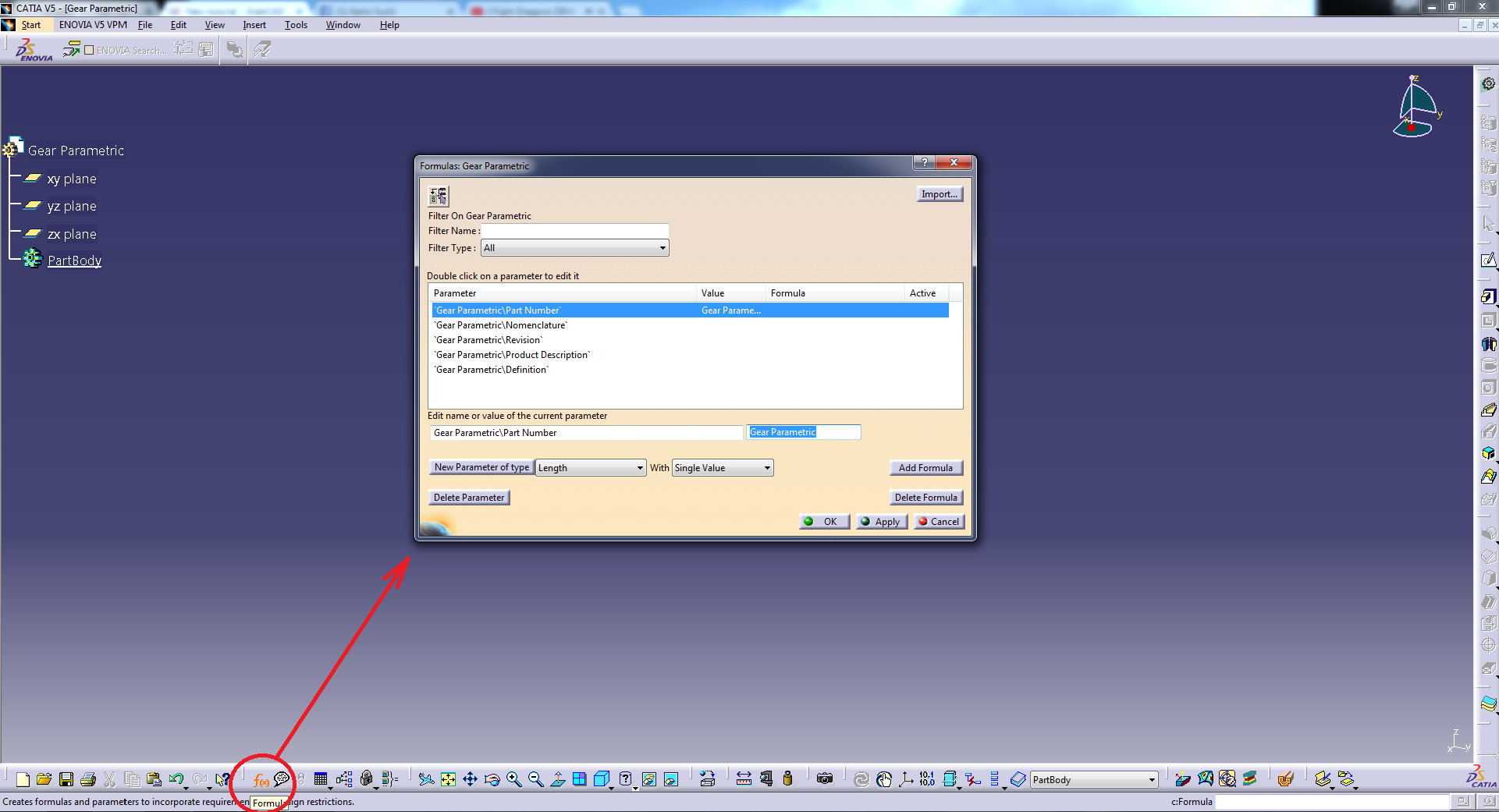Now you will open ˝formula˝ tool and you will change Filter type to Renamed parameters (just so we can focus better on our added formulas).

We are ready to insert formulas!

Number of teeth [n]

We change ´lenght´next to ´New parameter of type´ into ´integer´ and click ´New parameter of type´.

Rename Integer.1 to n

and add a start value of 25, press Apply to enter the value.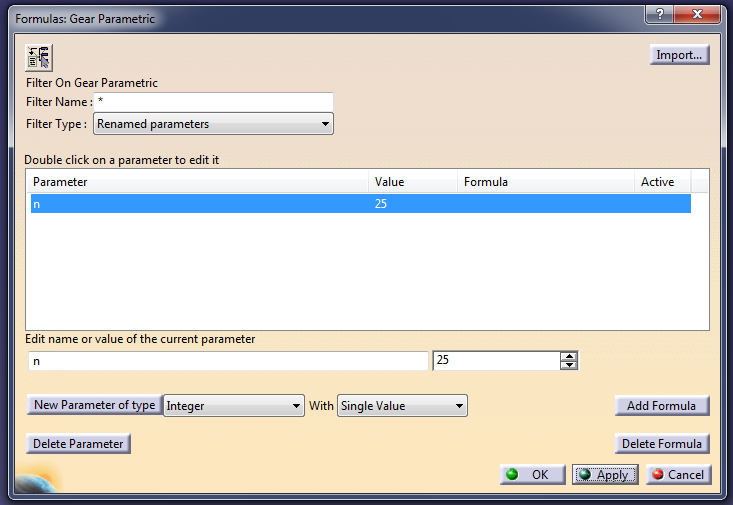Modulus of gear [m]

We change Parameter of type that is currently Integer into Lenght, and press New Parameter of type.

Now we change Lenght.1 to m, and add a start value of 6mm.

Again by clicking Apply we assign the given value.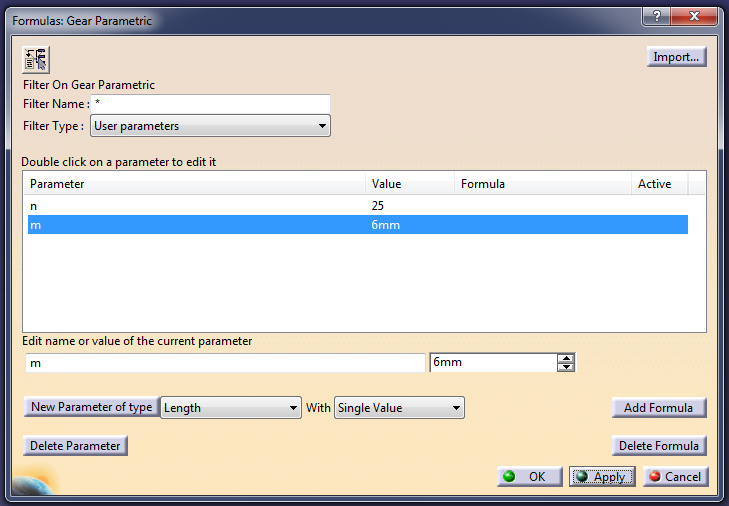Inserting angle values

We change Parameter of type to Angle, press New Parameter of type

We will call the first angle ´phi´ and give it a value of 45deg.

By clicking Apply we assign the value.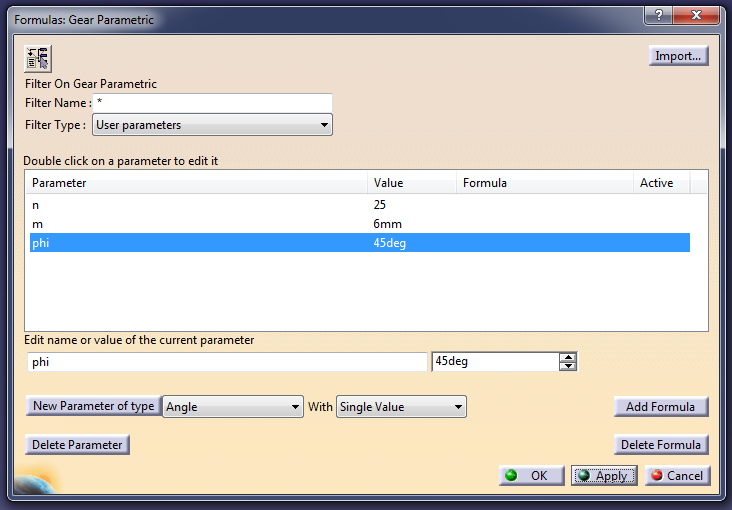We will repeat the same process for the other angle and we will call it ´alpha´

alpha = 20deg

Now we have to assign a formula to the new angle we will call ´psi´

We rename Angle.3 to psi, and instead of inserting a value in the box right next to it, we press the box Add Formula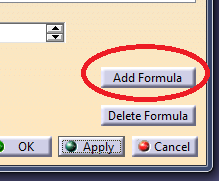We will write a formula ´360deg/n´, and press OK

(IT IS IMPORTANT TO WRITE ´deg´, OTHERWISE CATIA WILL NOT BE ABLE TO RECOGNIZE THE VALUE AS AN ANGLE)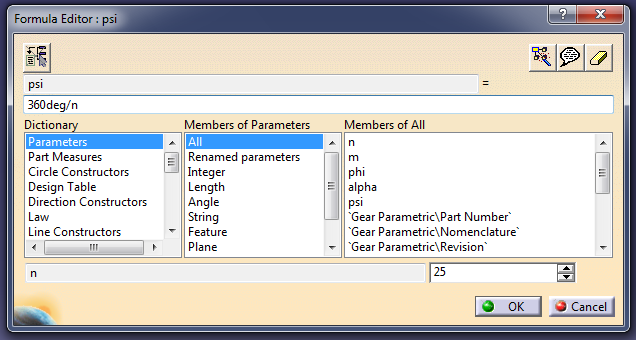We press Apply once more!

Now let´s go and enter Lenght as New Parameter of type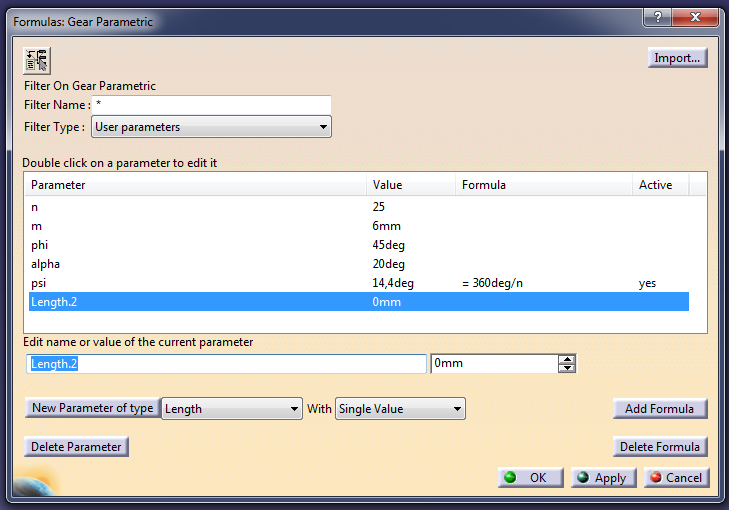We will rename Lenght.2 to Ro

And we will Add Formula to it

m*n/2

We click Apply once more.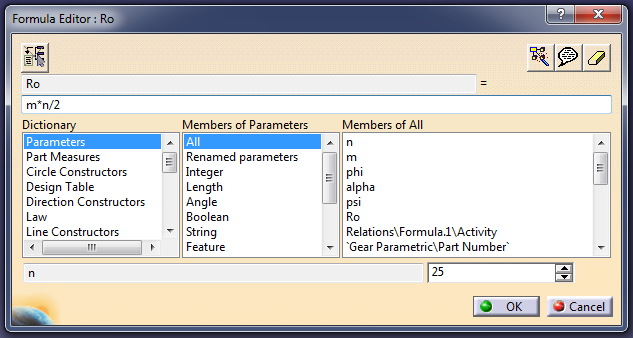Now you will enter the rest of parameters yourself:

Name of parameter: Ra

formula: Ro+m

Name of parameter: Rb

formula: Ro*cos(alpha)

Name of parameter: Rf

formula: Ro-1.25*m

Name of parameter: h

formula: Ro/2

(for h you don´t have to add formula, you can just enter the value you want or need) - only do this if you know what you are doing!

In the end you will have this: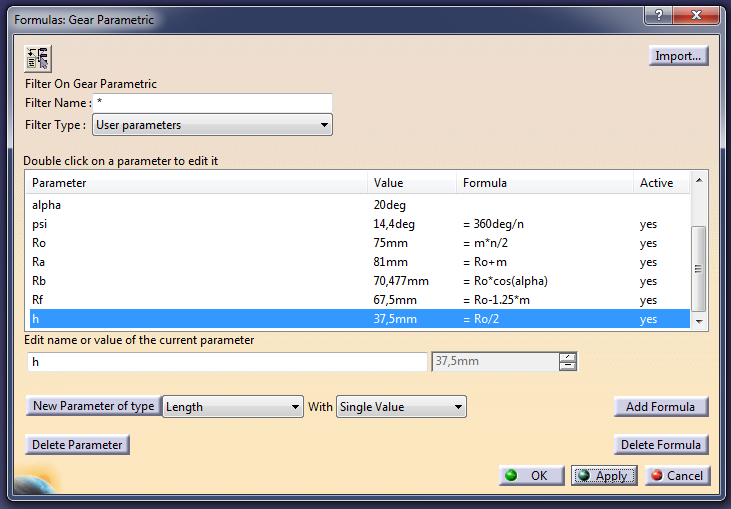Note that all formulas need to be active! (If there is no instead of yes delete parameter and write it again)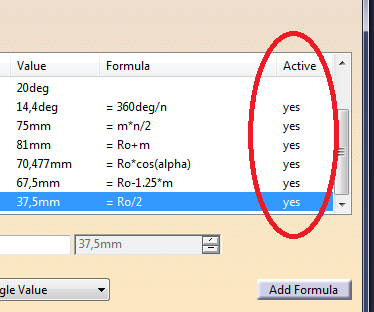We are done, you can exit Formulas: Gear Parametric by pressing OK.

2. ### Step 2: Modelling the gear

Now that we have our formulas we can start making the gear itself!

We will select XY plane and press Sketch to open the sketcher.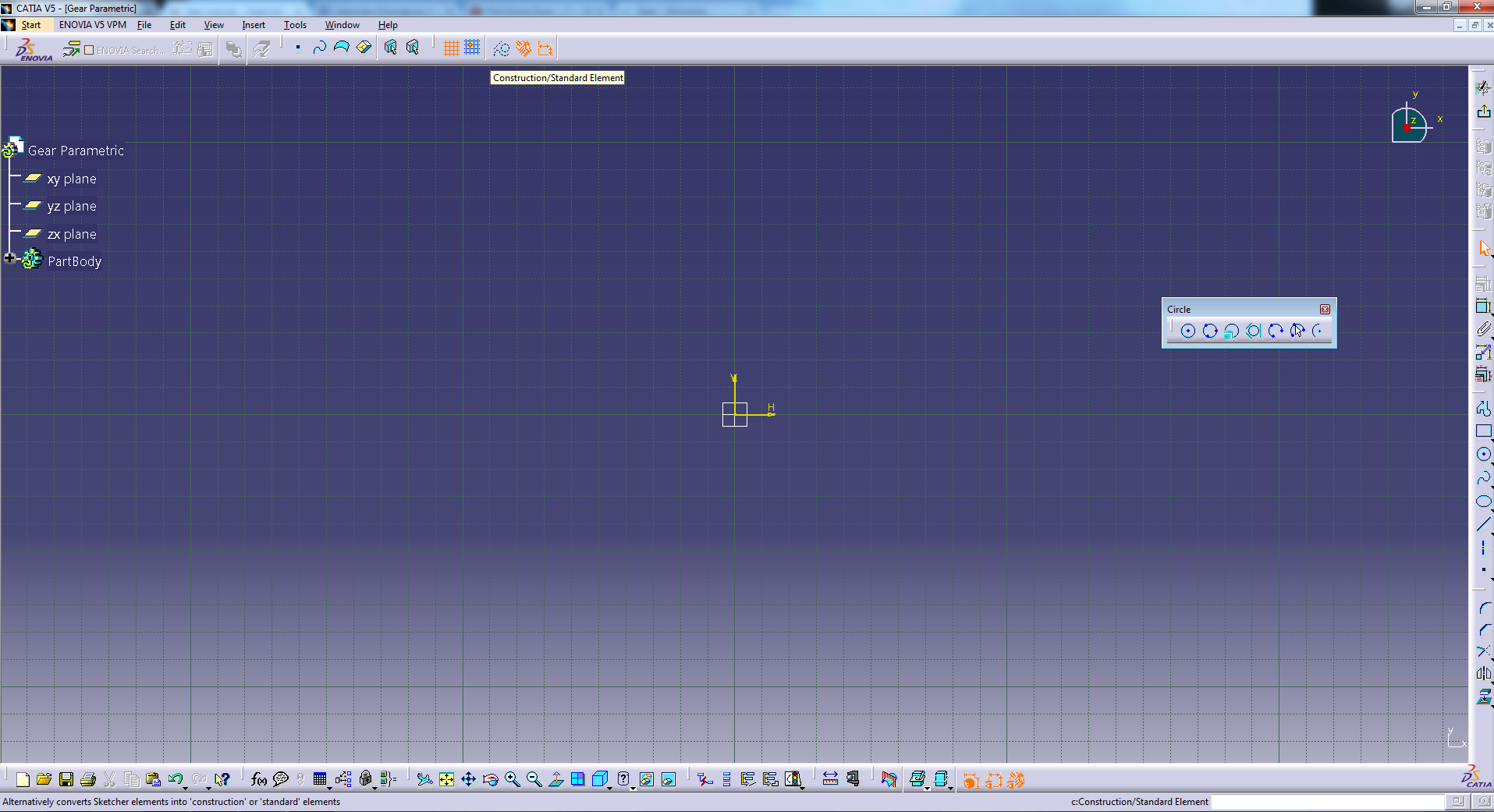We need to select Construction/Standard Element so it gets activated (orange in color)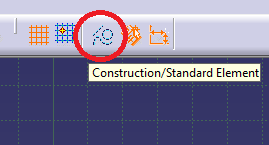Now we drag circle toolbar out so it expands for ease of access, and double click on the circle!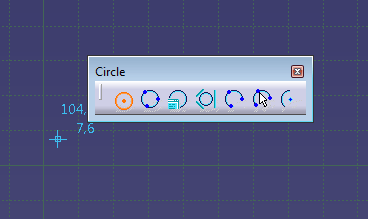We will draw 4 circles, each with the center point in the origin.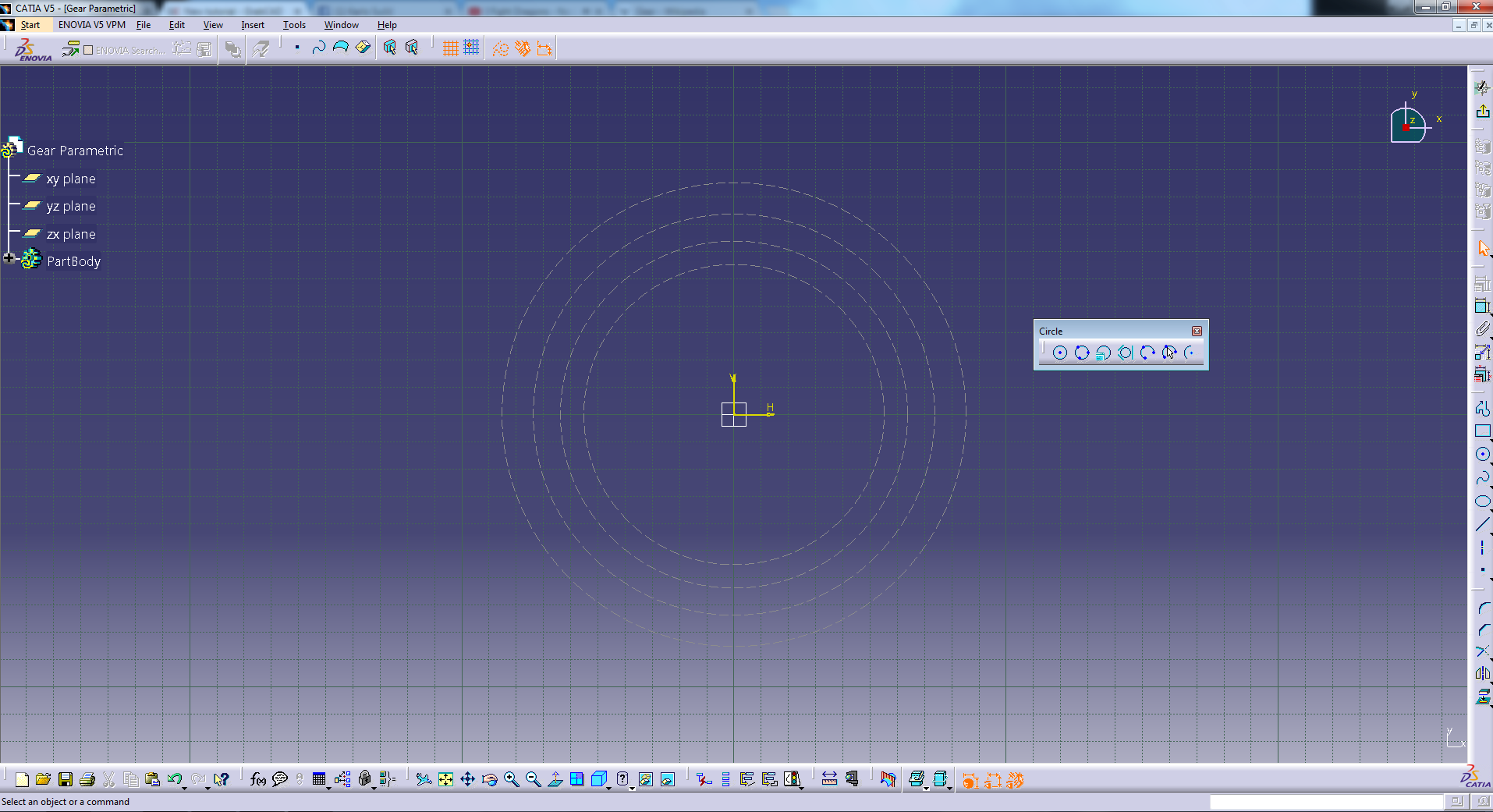We can start to constraint them using the Constraint tool.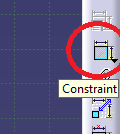To constraint the circles we click on them once, and change dimension to Radius!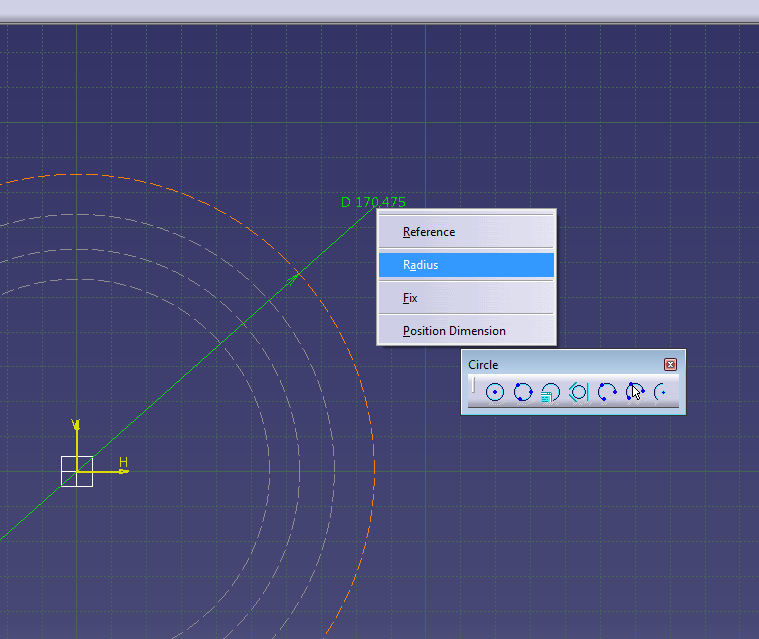You should be left with a dimension R

Right click on it and Edit formula like this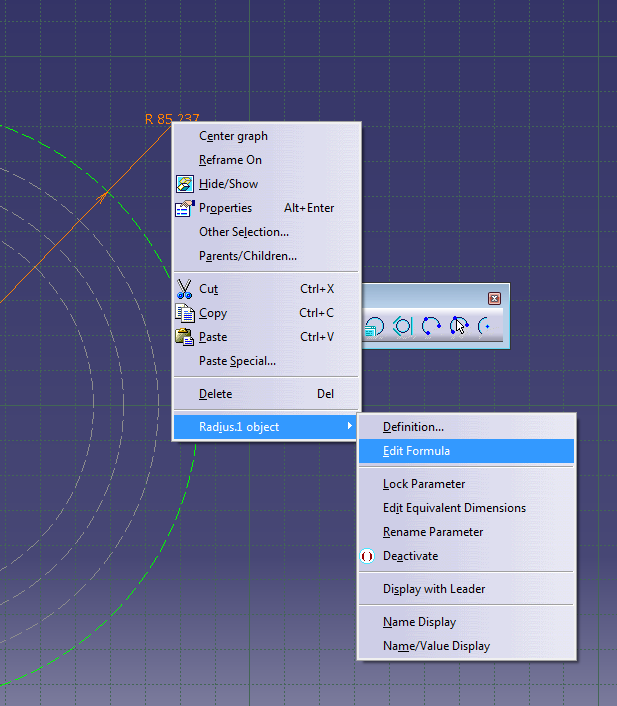Enter Ra as a value.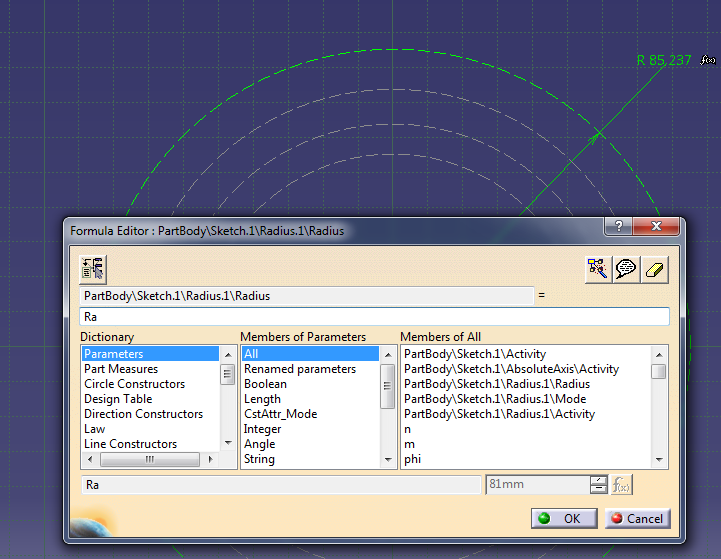Repeat the process for the other remaining circles, but instead of Ra enter

Ro

Rb

Rf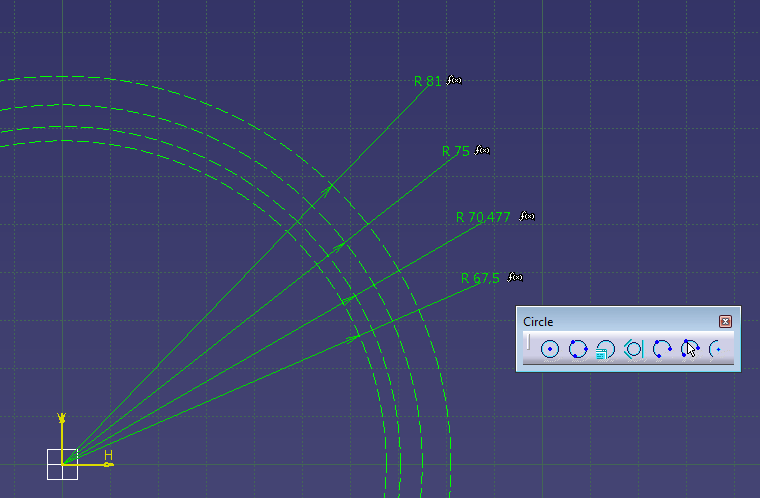Your values should be the same as mine, and there should be small f(x) icon next to them.

Now we select a line tool and we make three lines like this: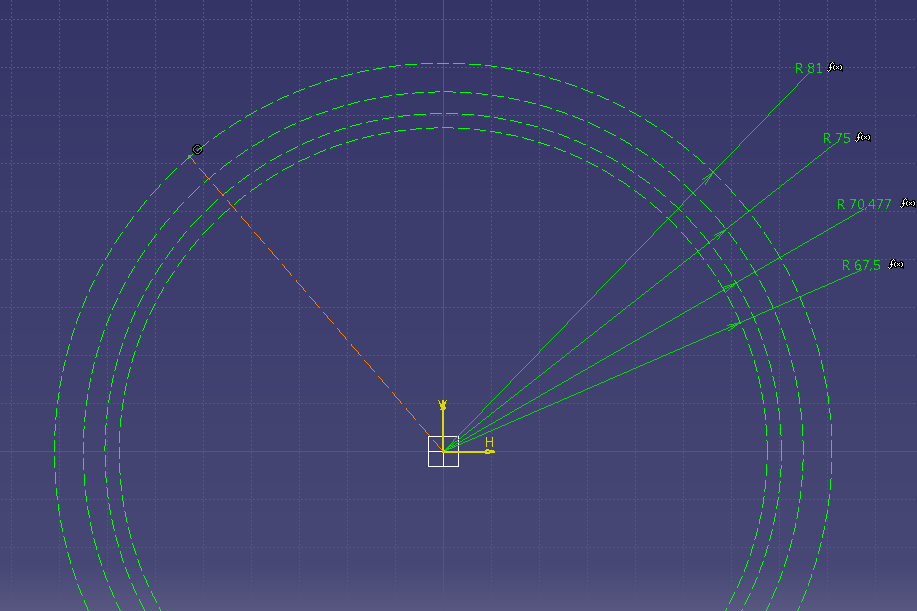First line is constrained to the vertical axis with the formula phi.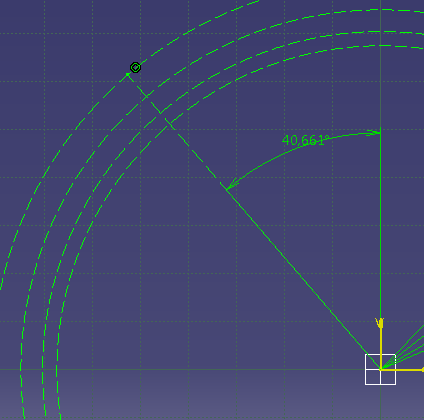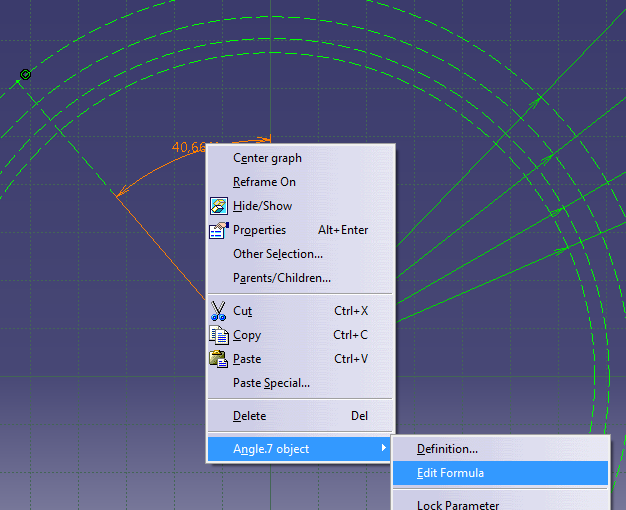Now we draw the second line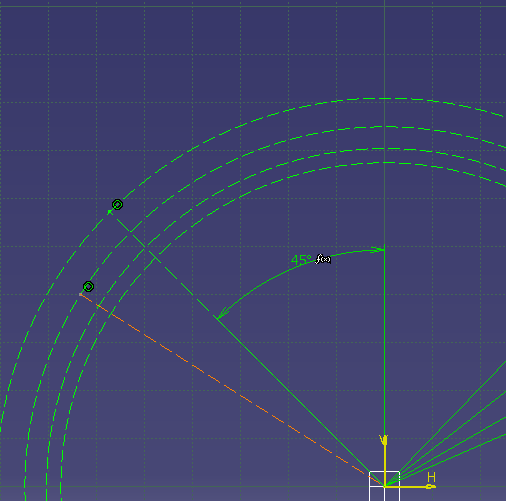And constraint it to the first one using formula psi/4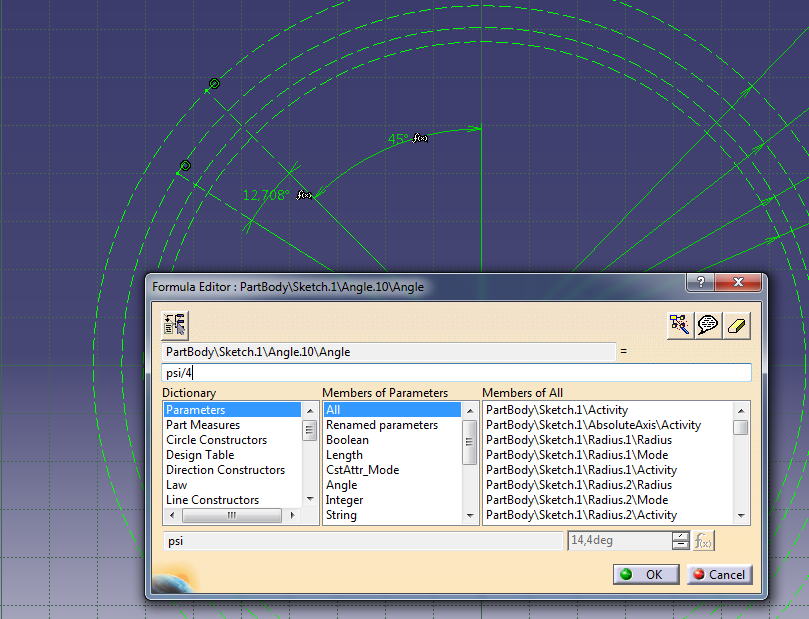We now draw the third line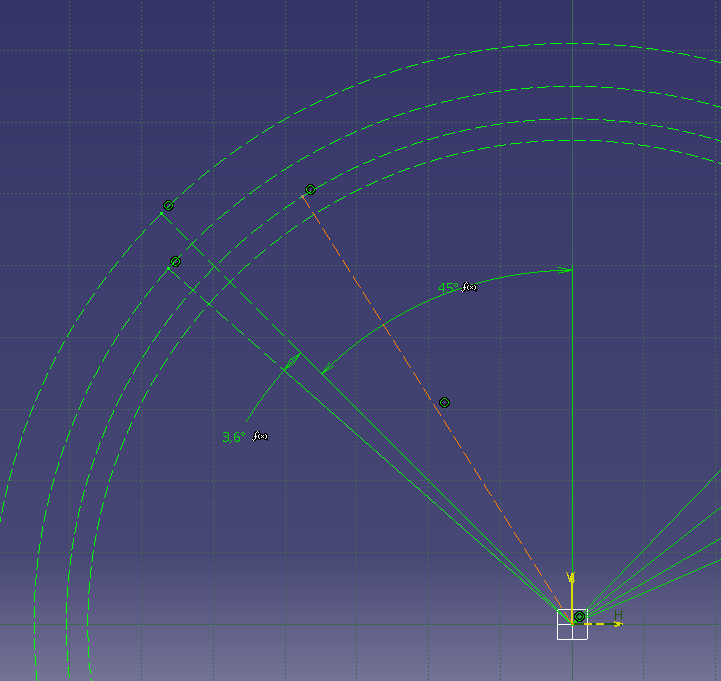And constraint it to the second line with the value alpha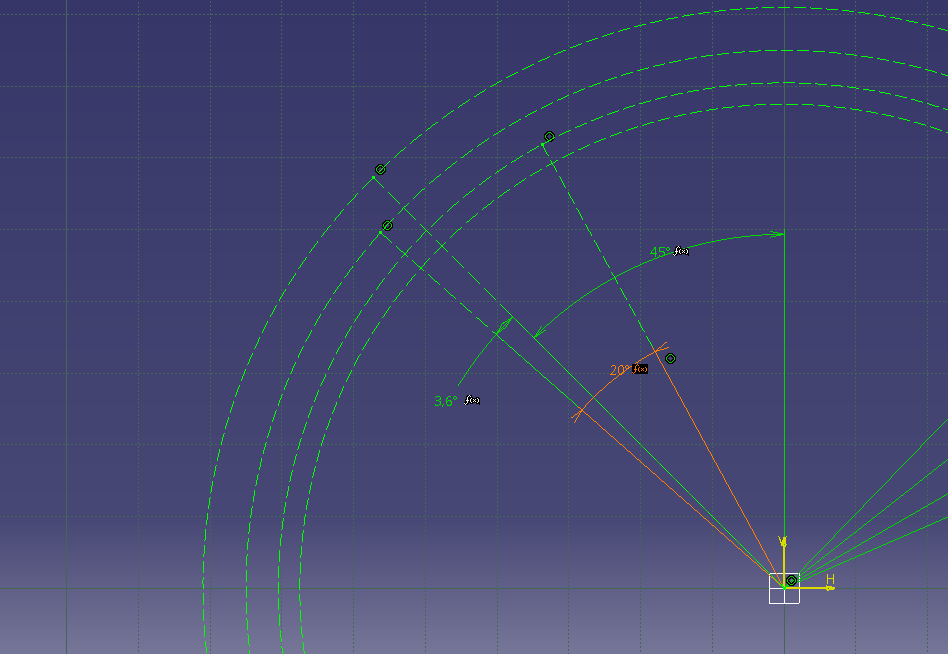Your sketch should now look like this: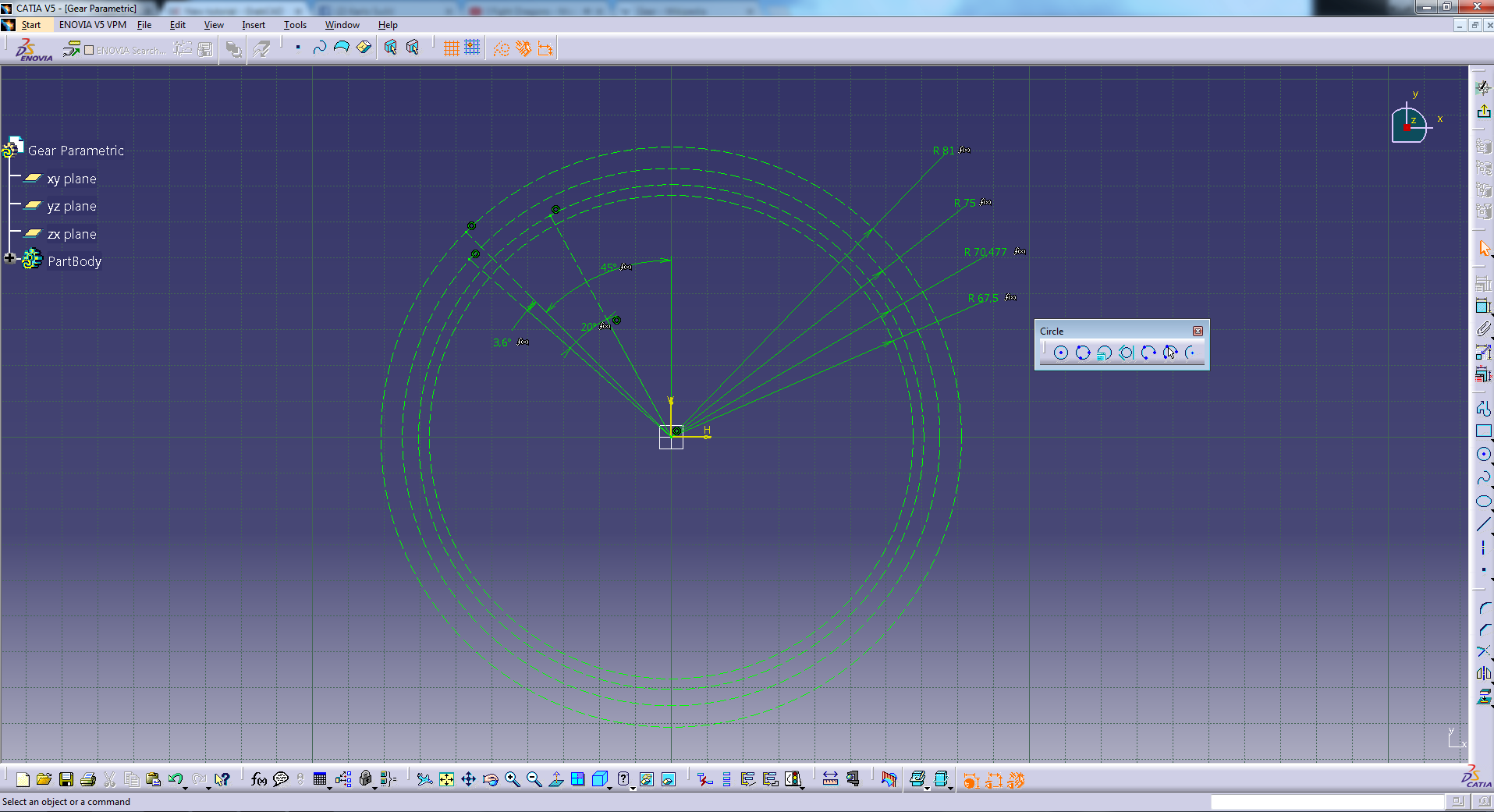We can begin to draw!

First we TURN OFF Construction/Standard Element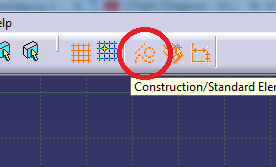Now we select the Arc tool from Circle Toolbar we dragged out for ease of access.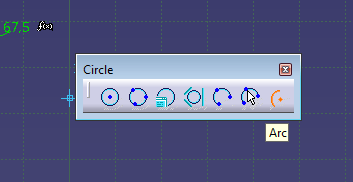And we select the end of our third line as a center of our arc by clicking on it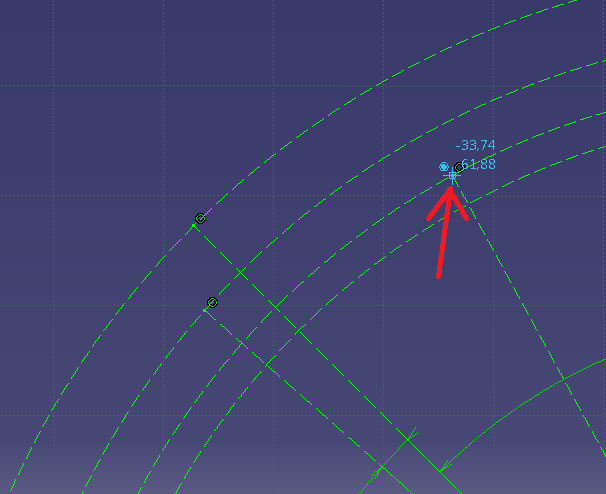We draw the arc by clicking the outside of our top circle, and inside of our Root circle like this: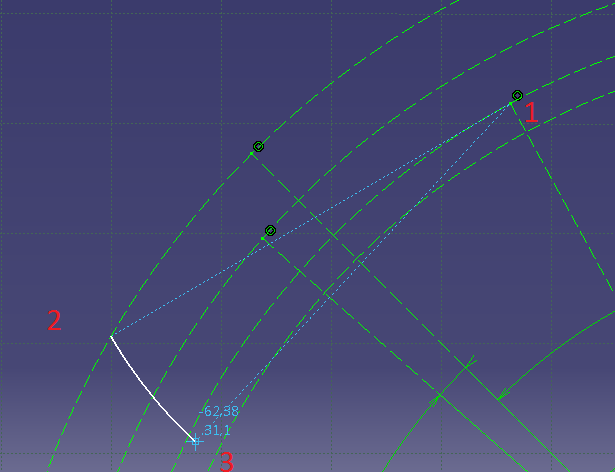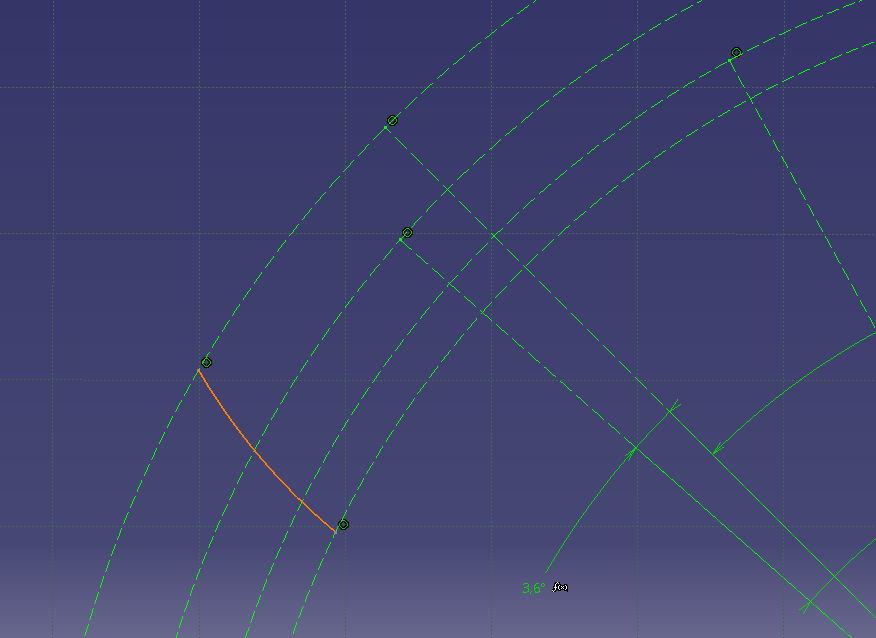BE CAREFUL SO THIS DOES NOT HAPPEN!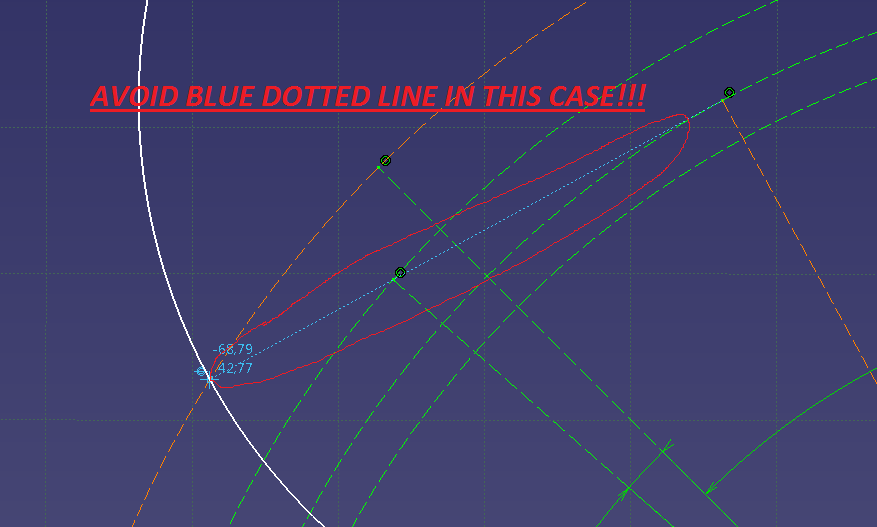Now let´s constraint the arc to the end point of our second line.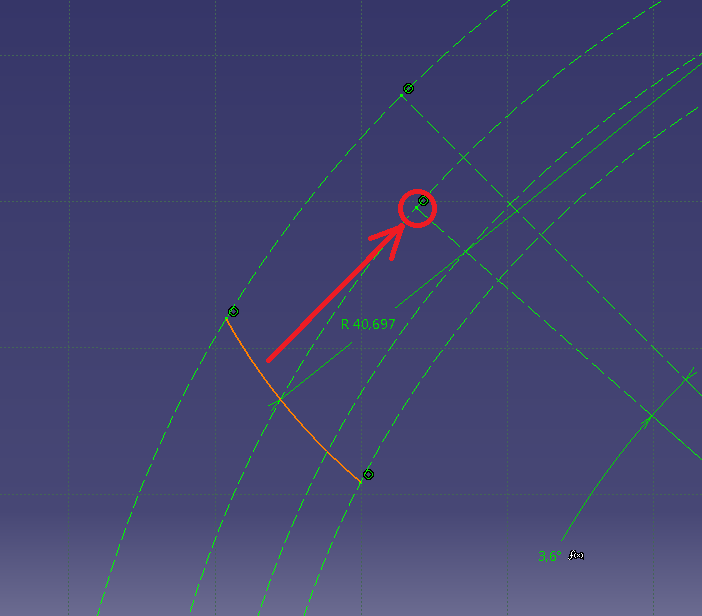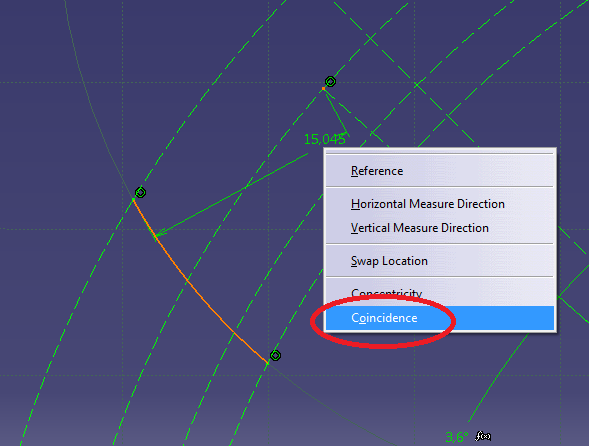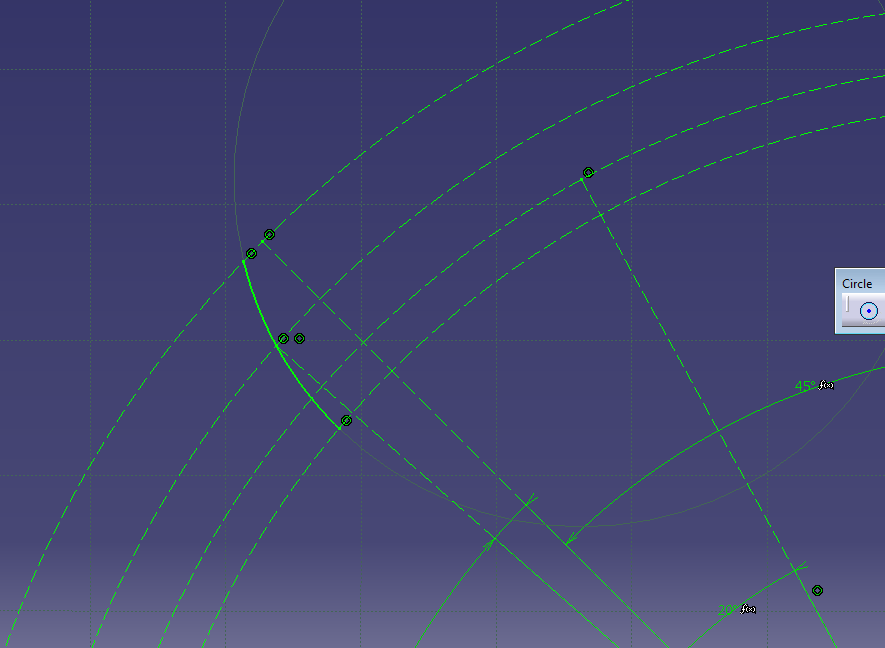Now we need to make a corner using a corner tool: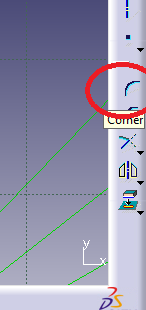Click on the arc, and then on the Root circle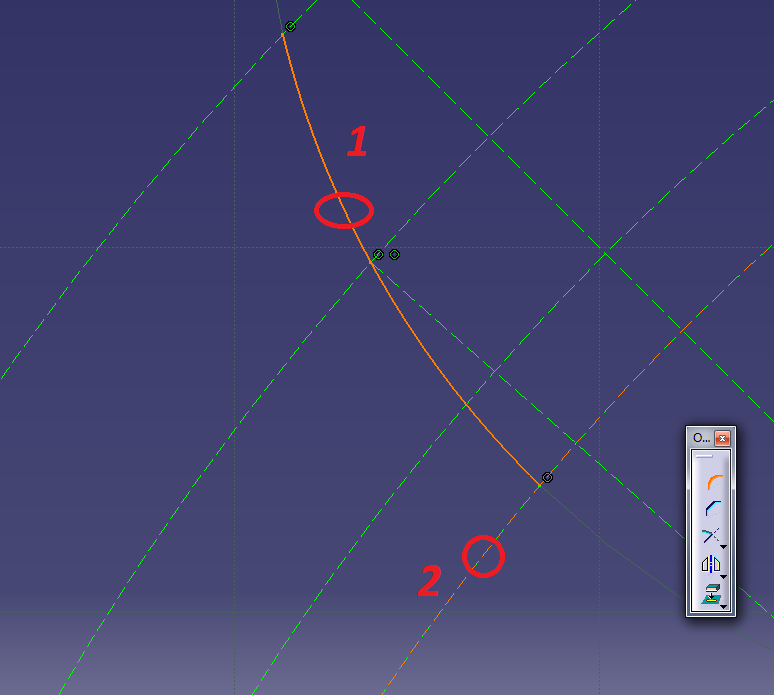You will get this: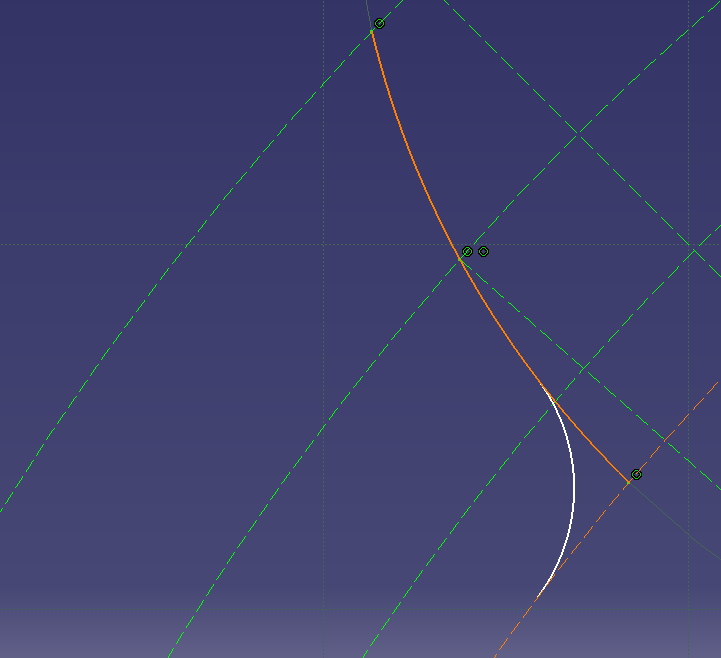Constrain that corner with the formula

0.35*m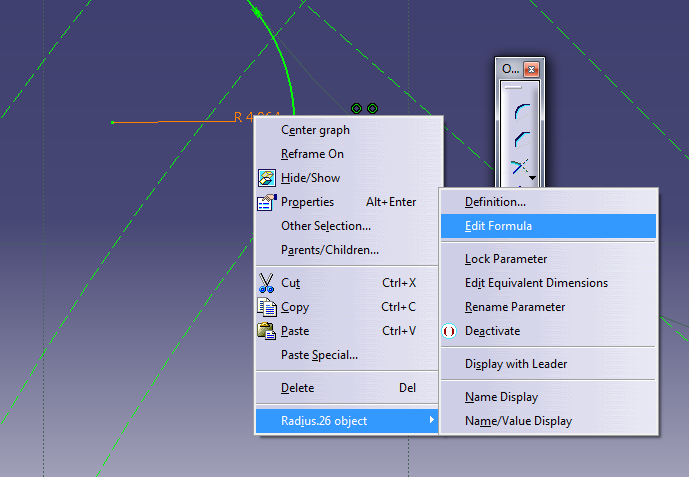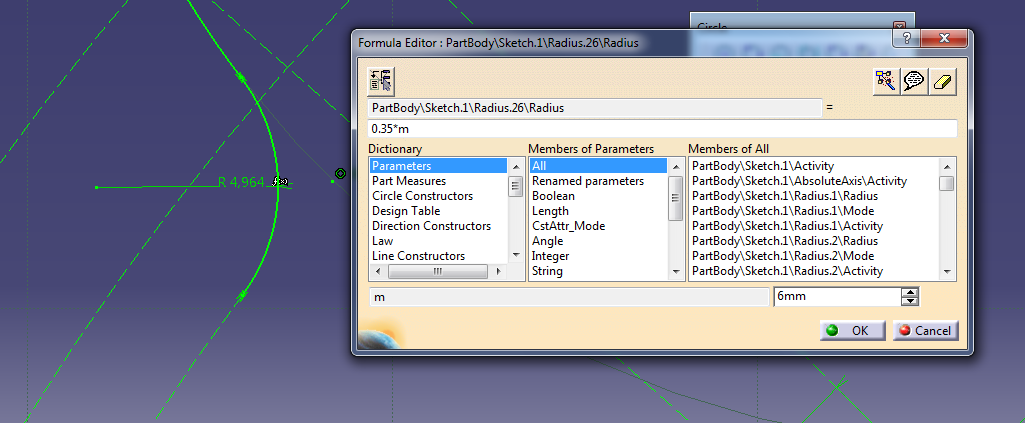Now we need to mirror the elements so while holding Ctrl we select corner and the arc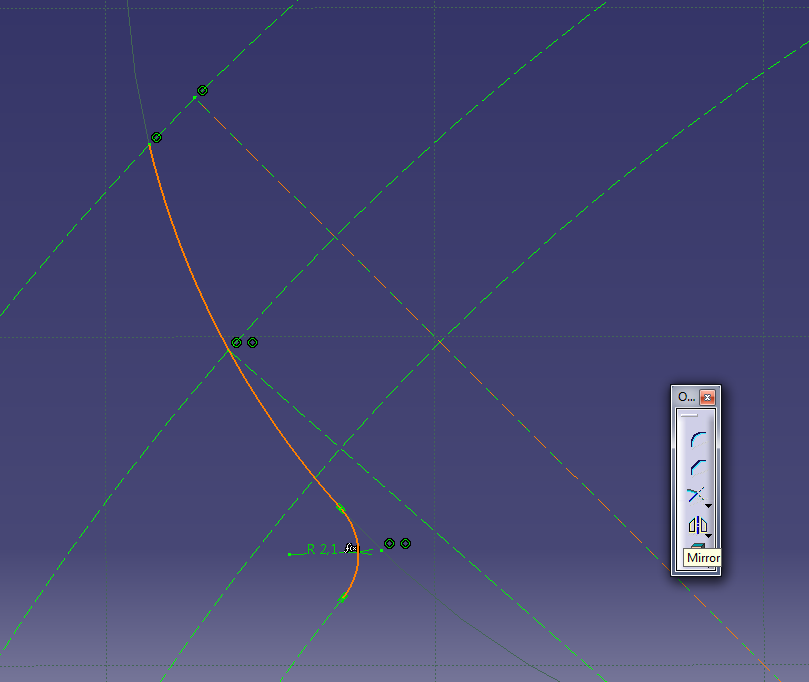We select the tool called Mirror and mirror the arc and corner using the first line as a center axis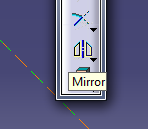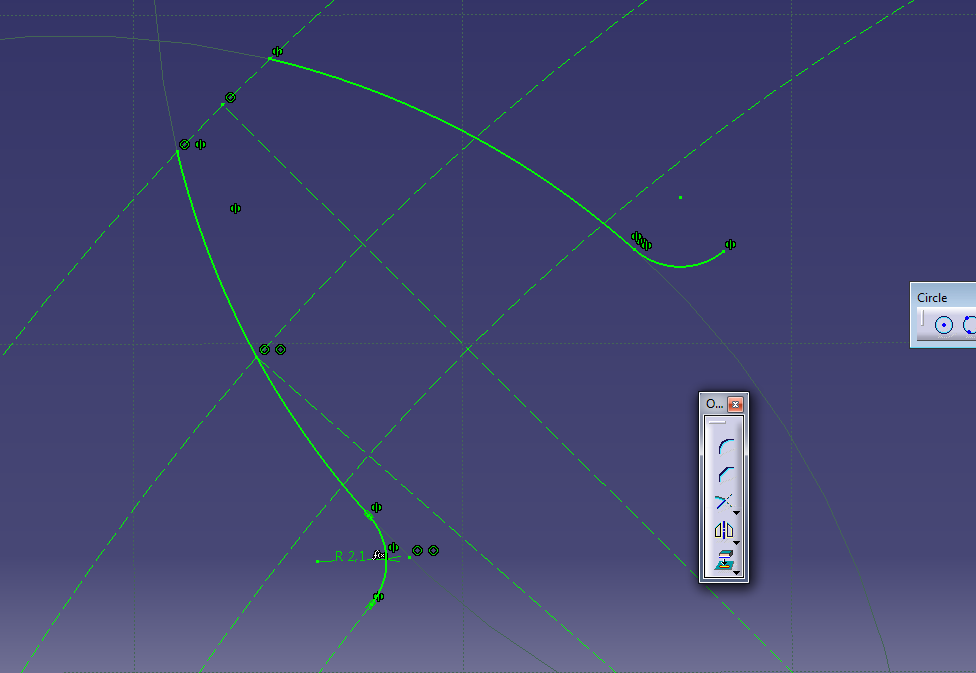We can see that the root circle is now broken, so we need to use constraint tool to connect it to the mirrored corner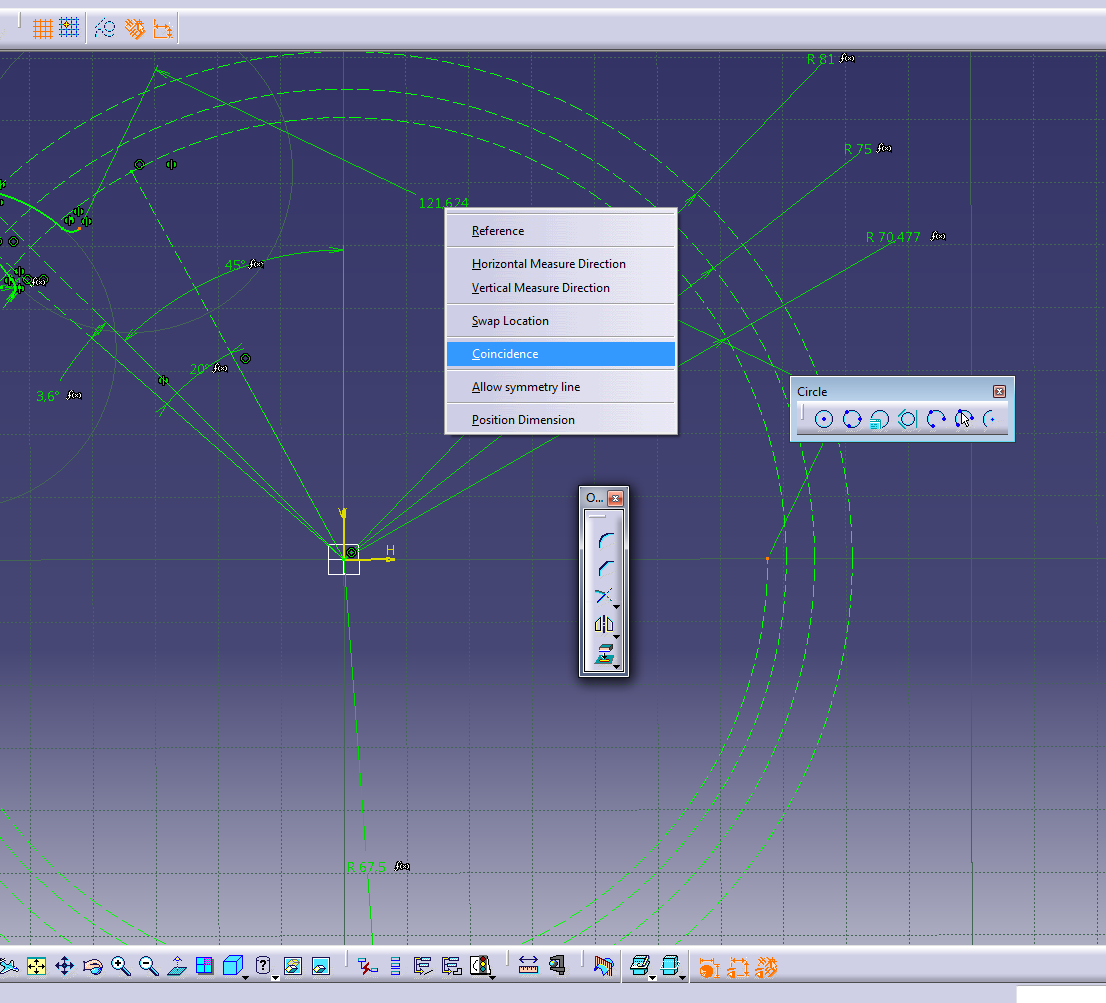We need to close the tooth sketch by using Arc tool, and use origin as the center of our arc, and corner endings as end points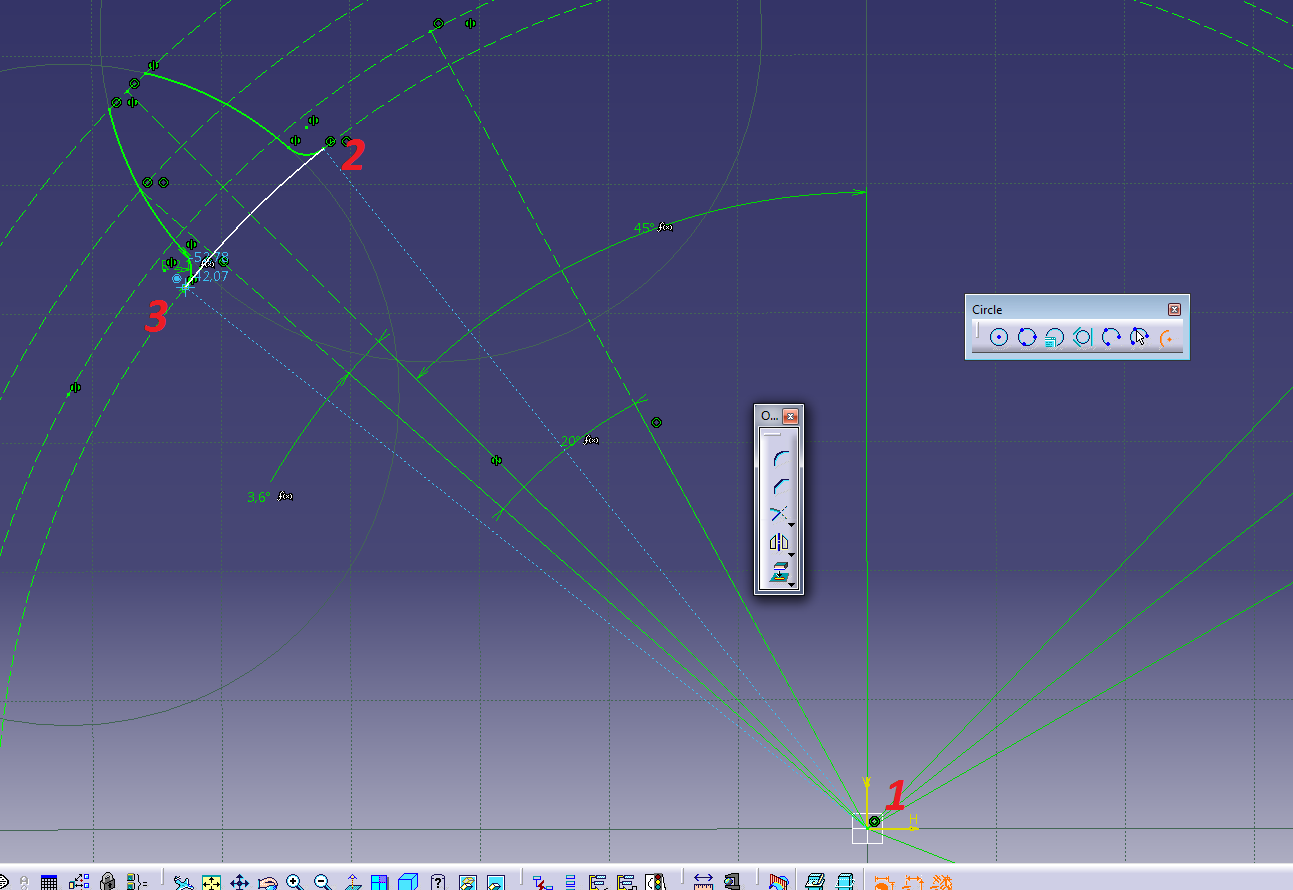That was the bottom, now we need to close the top using origin as our center point again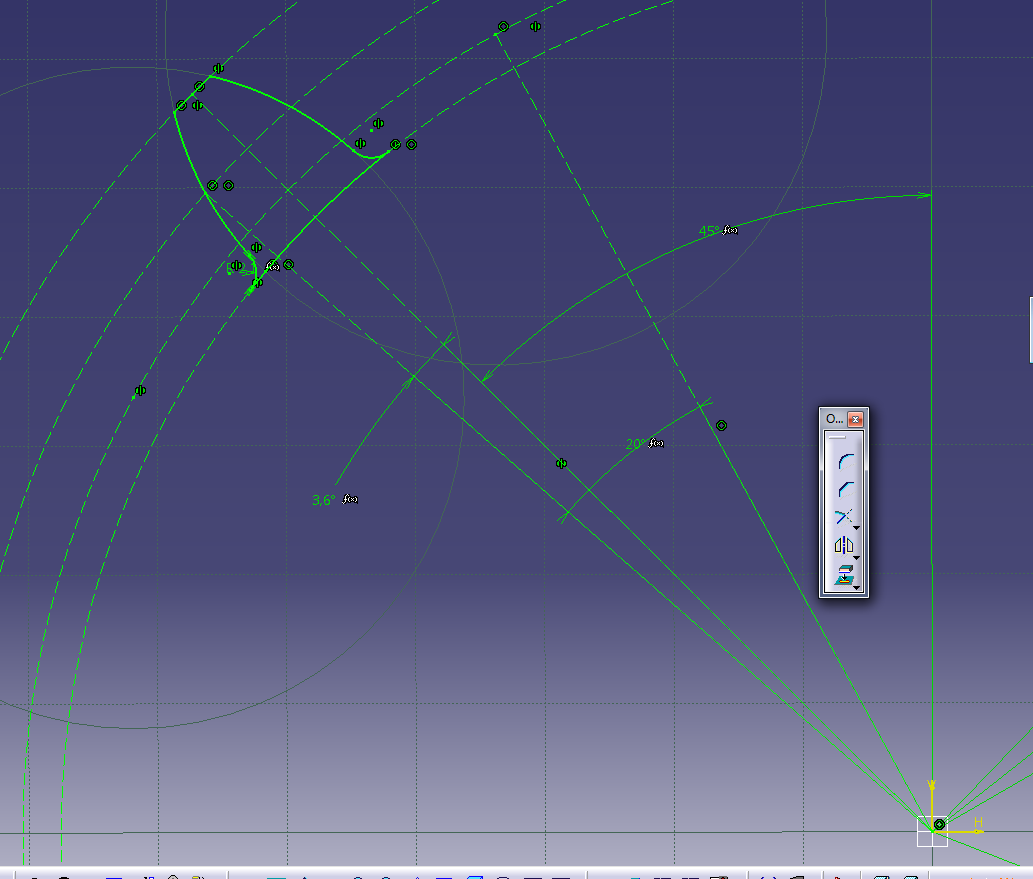We can finally exit workbench, and go to Knowledge Inspector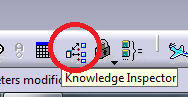And we will change the value of phi to 0 deg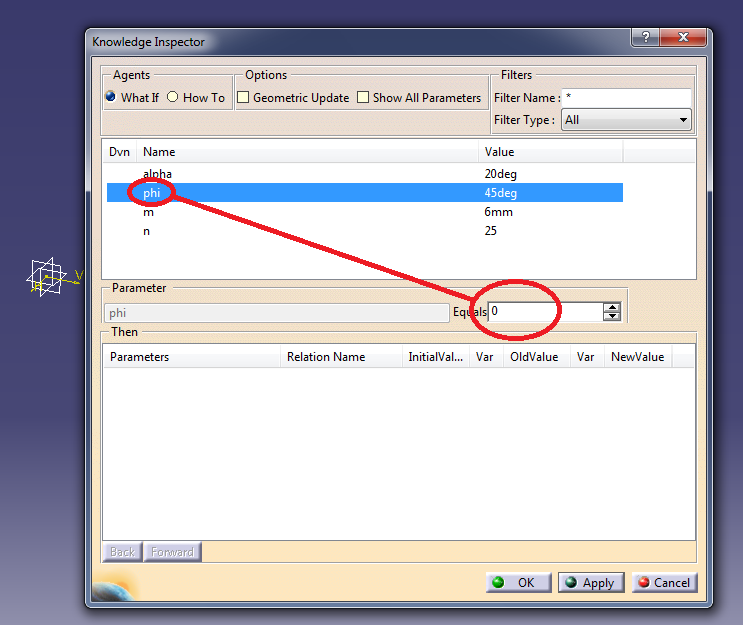Apply-->OK

Our tooth profile should move 45 degrees and stay as it was, if it does not, and this happens, you need to close the tooth profile again!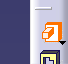And choose our tooth profile to be extruded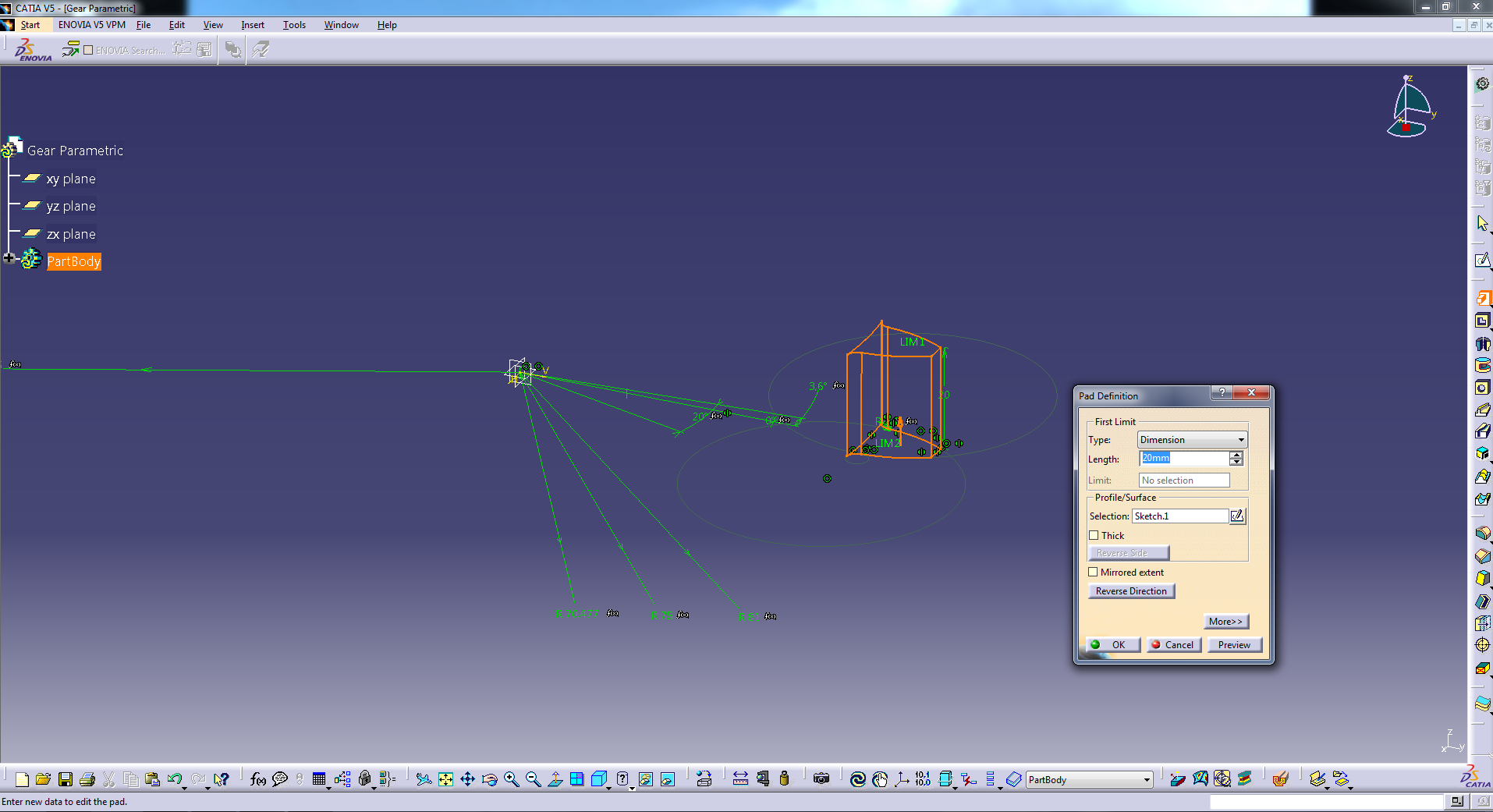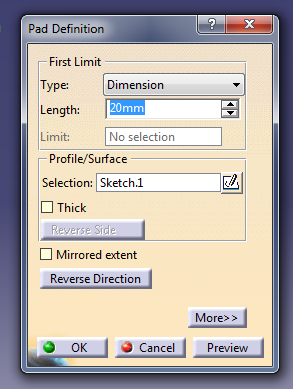We right click on the Lenght box that says 20mm currently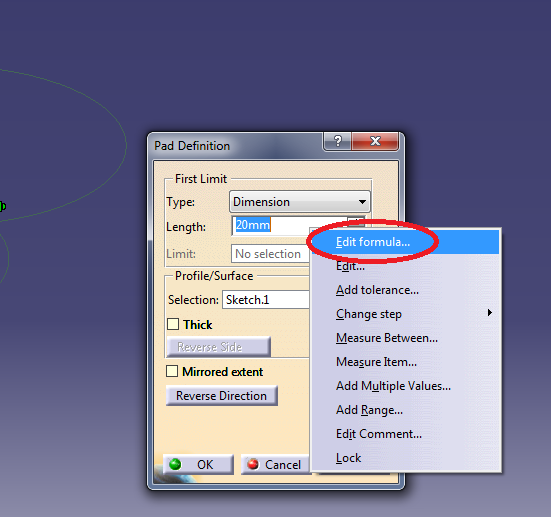And we enter h as our formula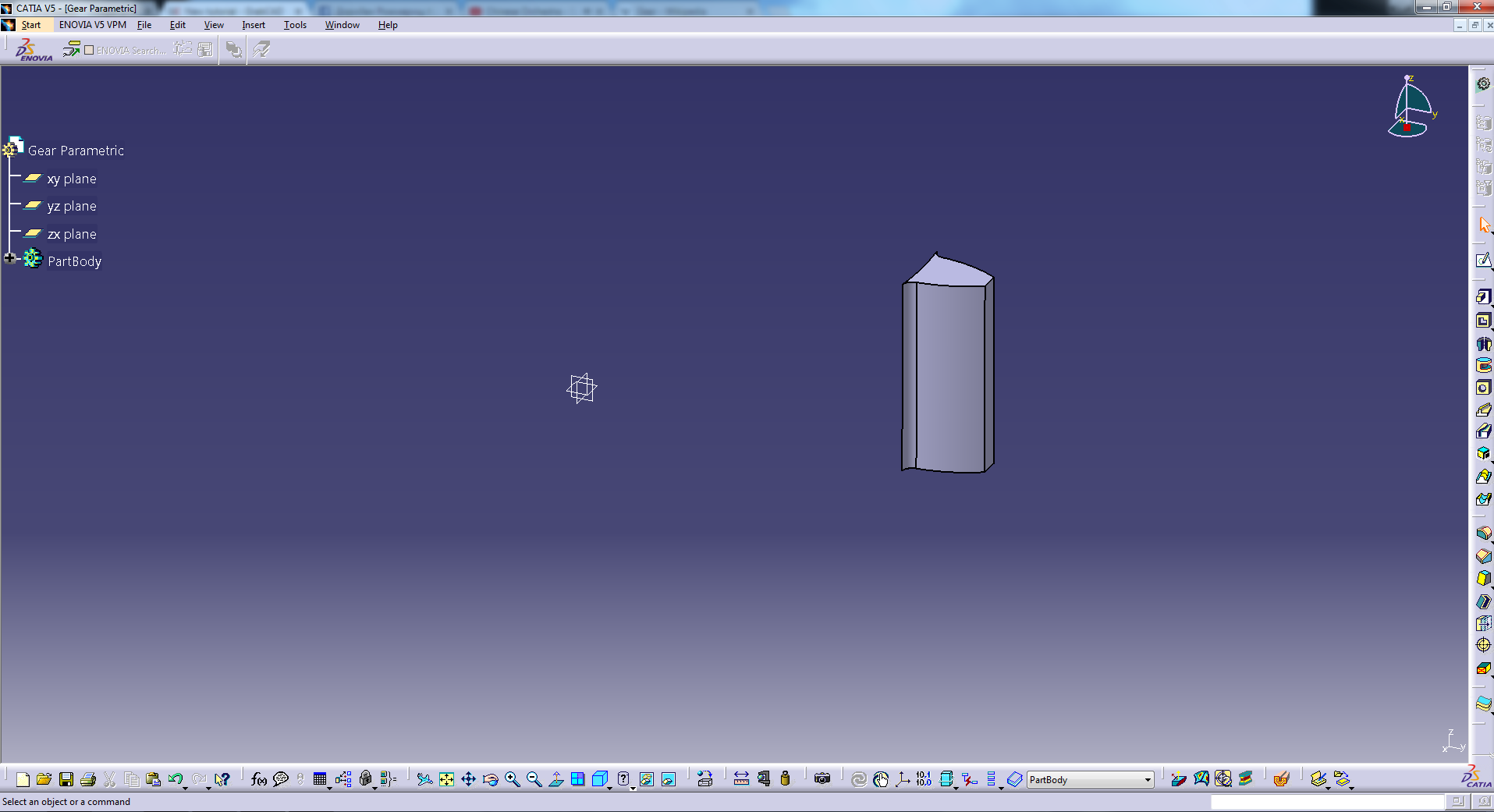Then we want n number of teeth so we choose circular pattern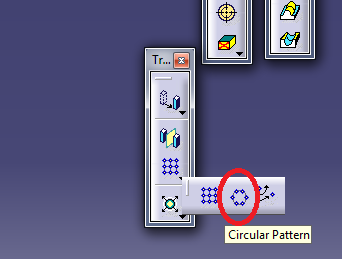Now we edit the values with right click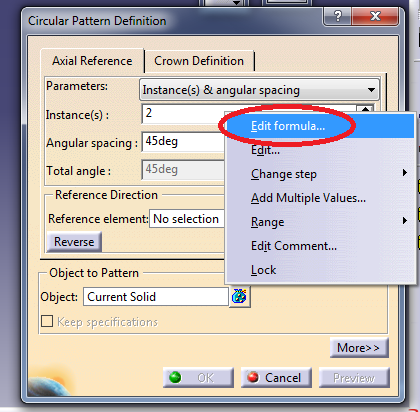Instance(s):-->Right click-->Edit Formula-->Enter n (number of teeth)

It should look like this, then edit Angular spacing the same way and enter psi in Edit formula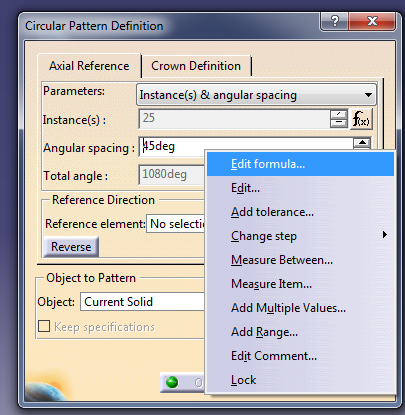Right click on Reference element: and choose Z axis in this case.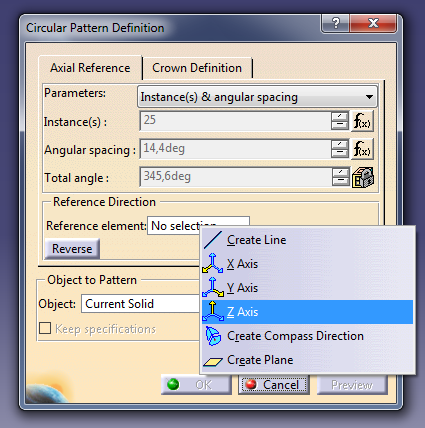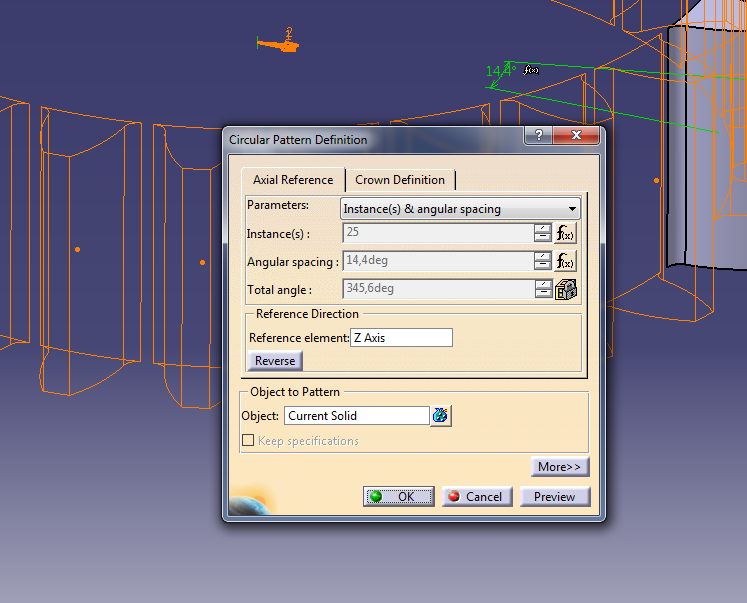Let´s fill the inside of our gear as well

We choose the plane we were working in (in this case it was XY) and draw a circle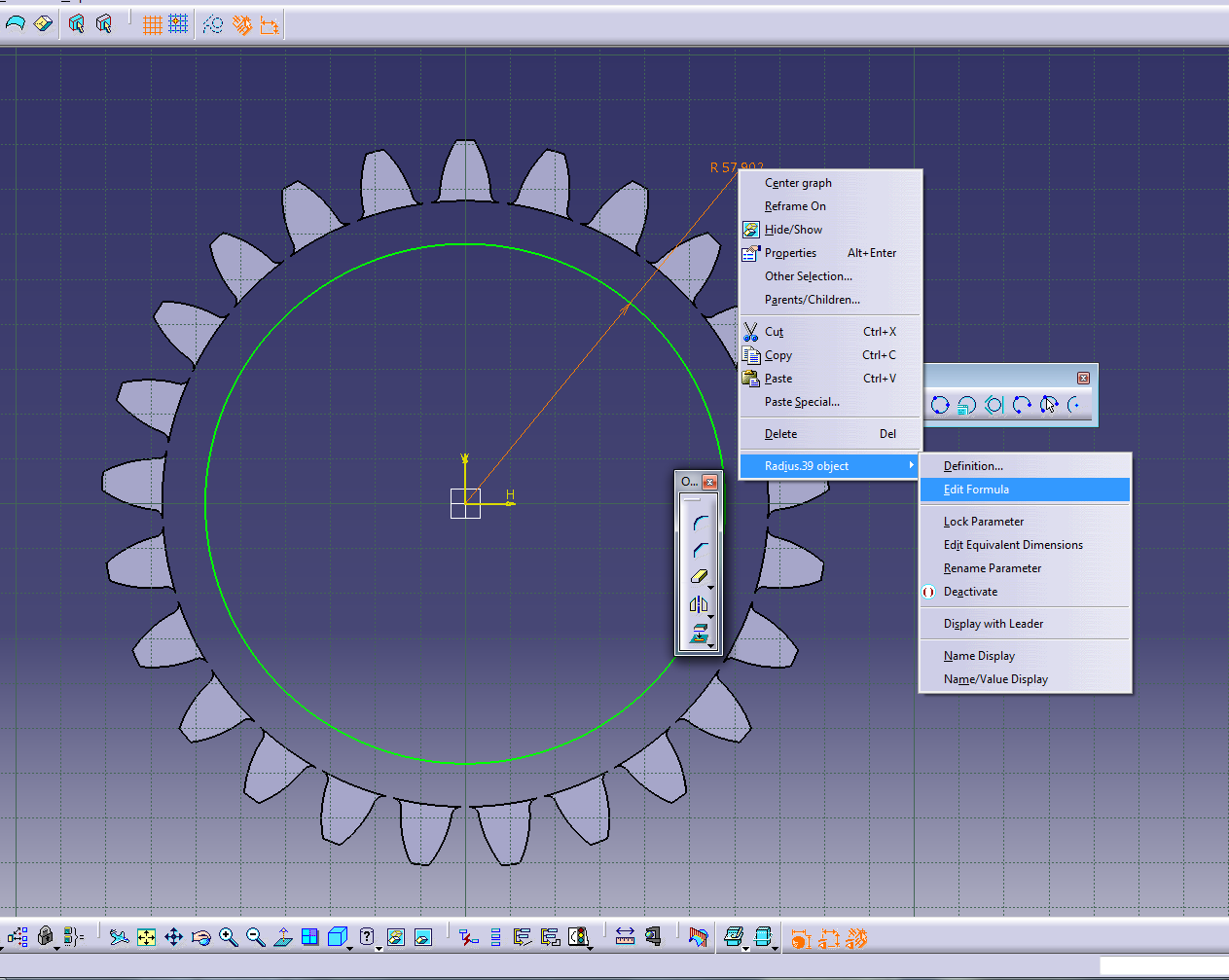Edit formula:

Rf*1.001

This is so our gear doesn´t ˝explode˝ while giving it different teeth and modulus values!

We exit the workbench and select pad to extrude the circle for the height of h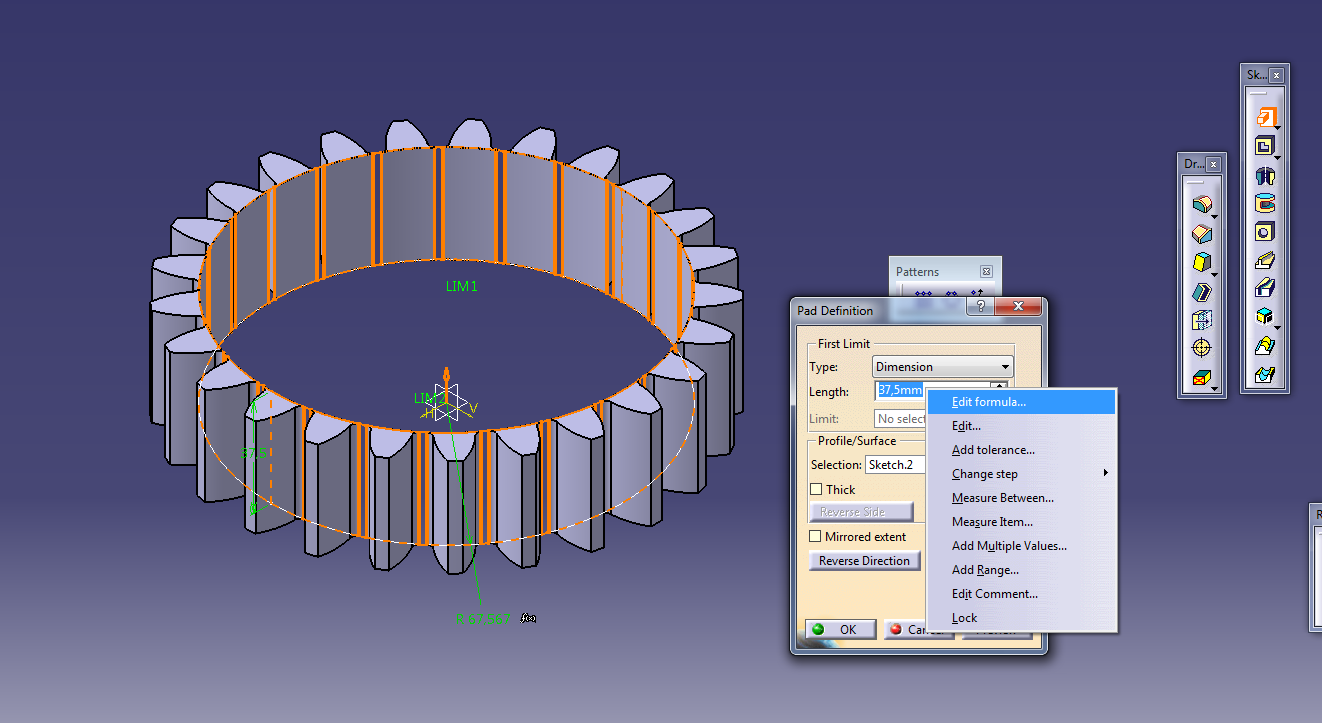We then press OK, and our gear is complete!

If you want to change number of teeth, modulus or height go to Knowledge Inspector and change the values.

Number of teeth (n) cannot be less than 17!!! Tooth profile will be deformed!

n=25

m=6mm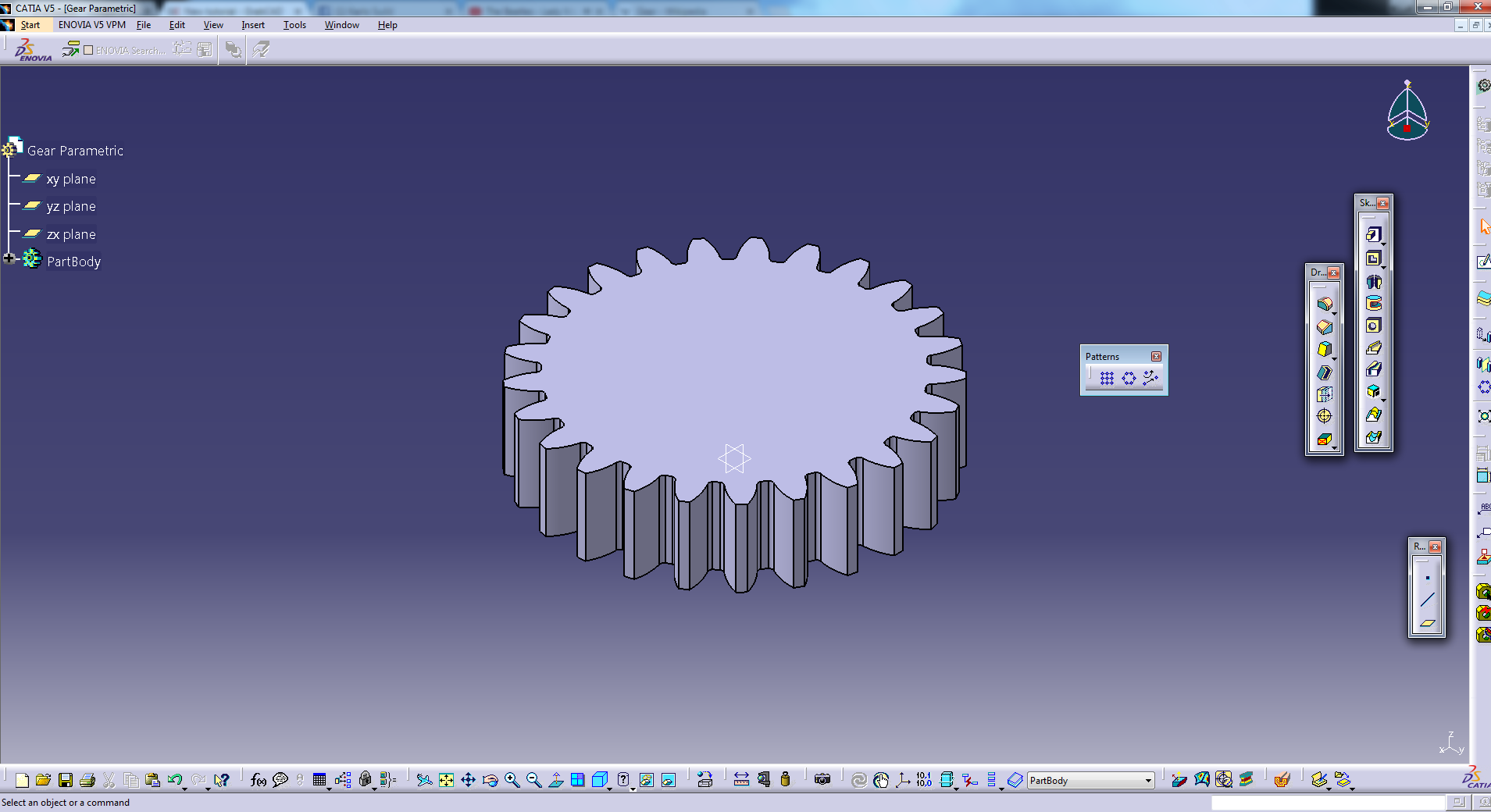Now we perform the so called Cross check

n=71

m=4mm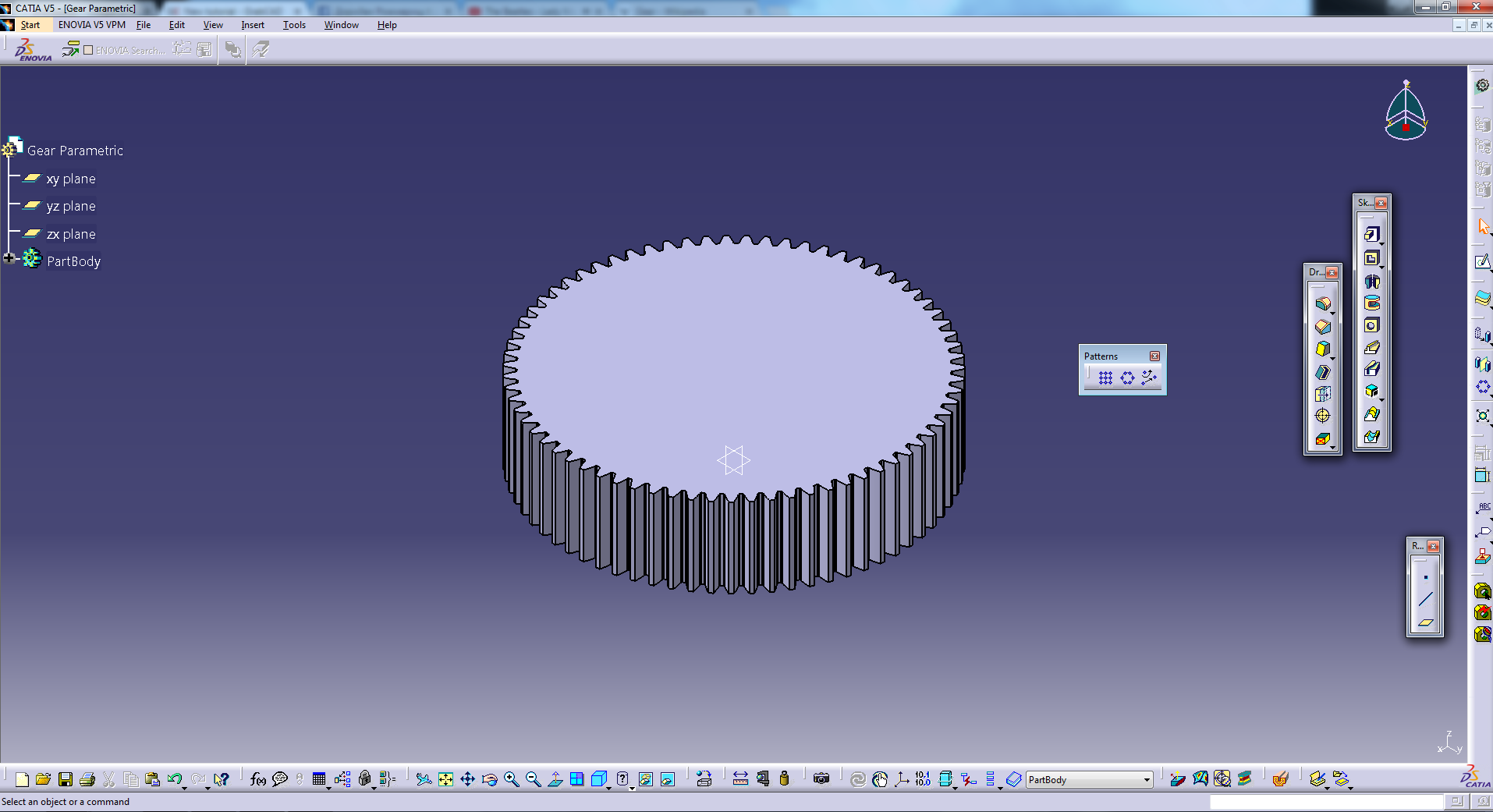n=17

m=8mm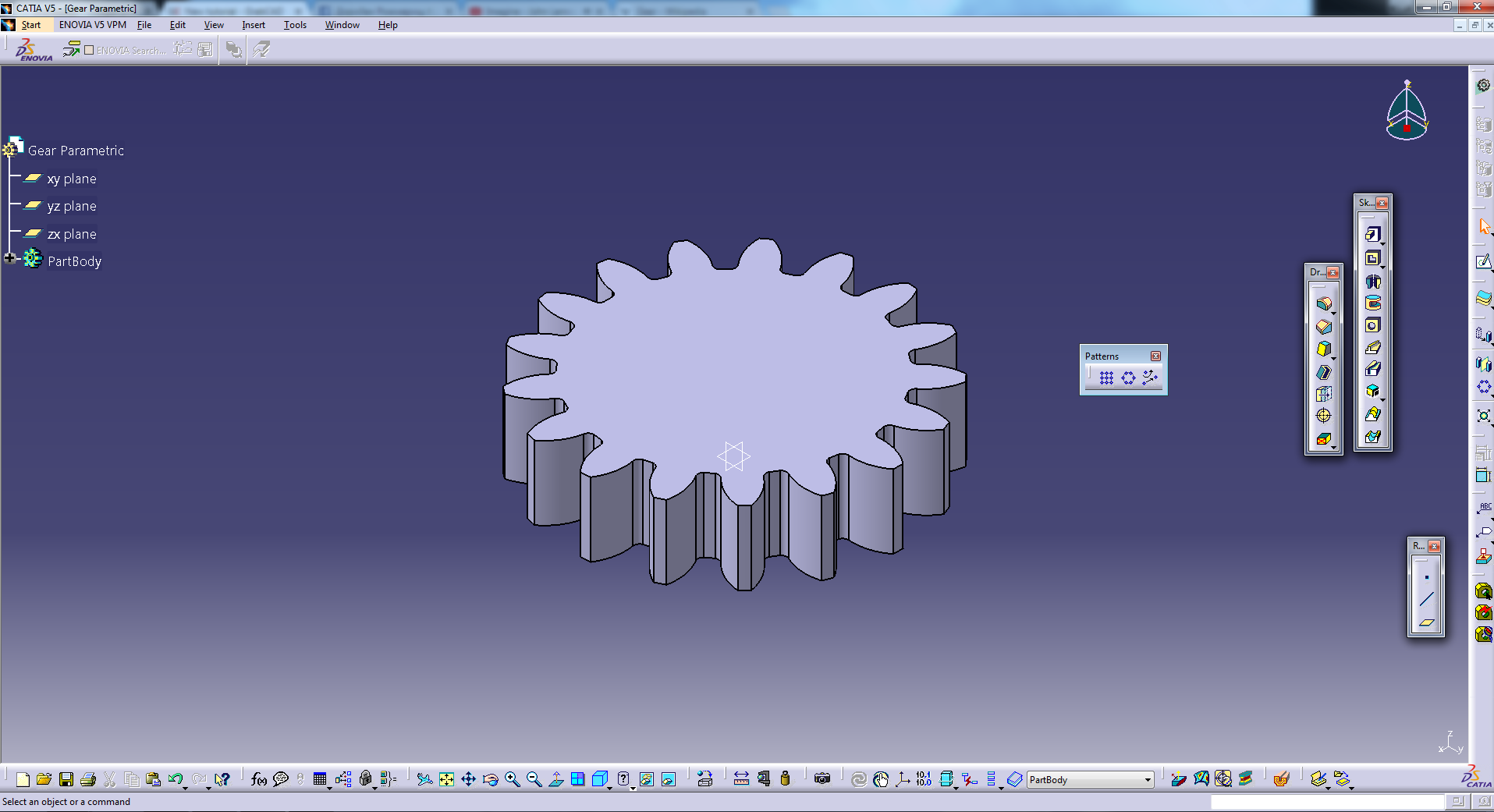If the cross check works without problems, your gear is done!

Ctrl+S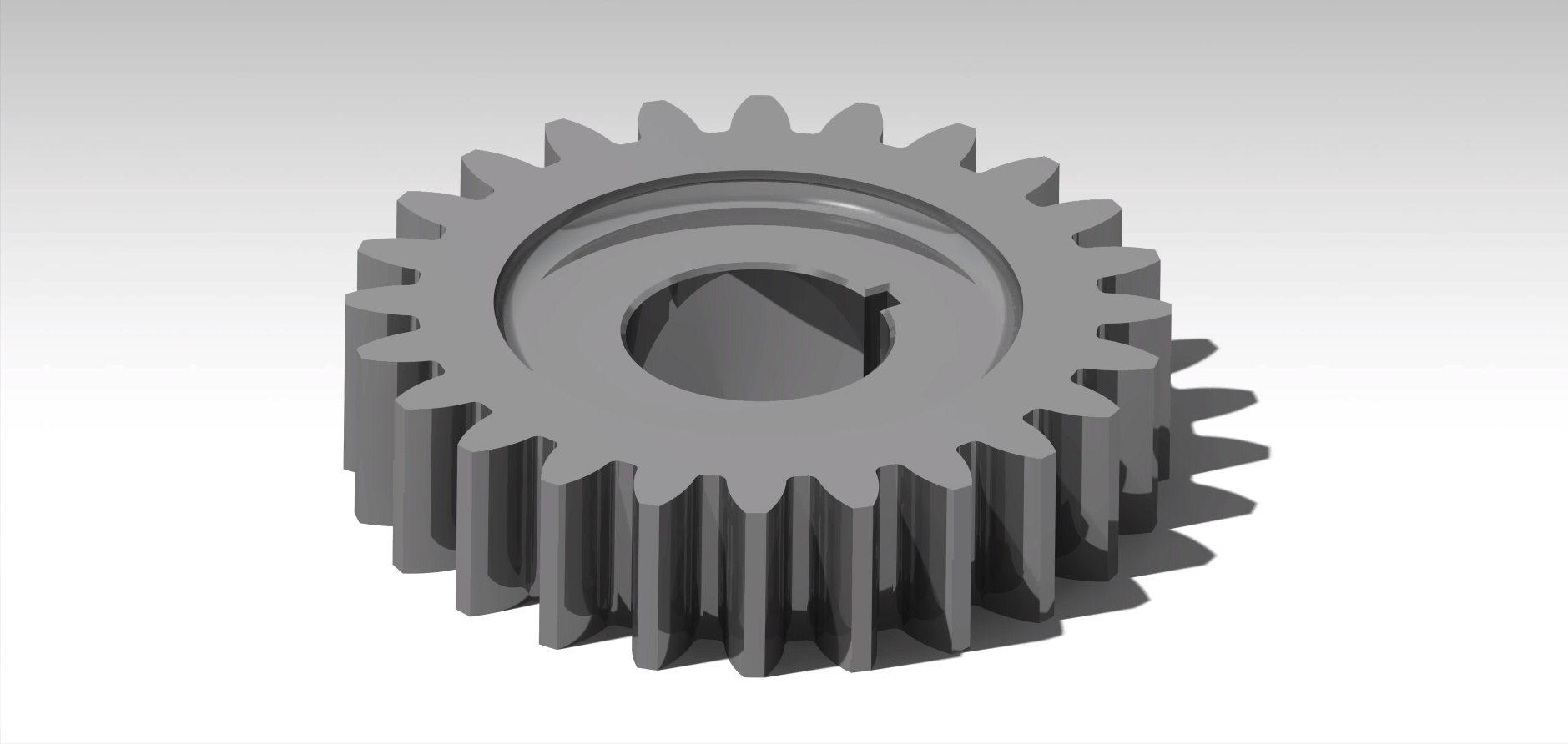﻿